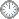# “执行定理”的证明(c)

This is an in-mail from TYUST.
新入の者--> What is going on ? (redirected)new

(接前: 31 30 28) “执行定理” (Th1.6) 的证明(c).
.
* 之前的温习中看出这么一条:
A ~ very ample, A ≤ r  <==> X ∈ 有界族.

.

(所谓概念化, 就是用特定名词“封装”若干条件).
.
* 证明的第二段往5.9靠拢, 凑出3个条件:
---- H' ~ 有界重.
---- H' - B' ample.
---- (X', B') eps-lc. (?)

(原作所谓的替换, 即“代入”).
.

.
Let C = 1/2A. Then we have lct(X, B, |A|R) = 1/2 lct(X, B, |C|R)...
---- 减半的 A , 对应加倍的 lct 值.
---- 添加系数 1/2 达成相等.
(此手法的好处是什么呢 ?)
.
..hence it is enough to give a positive lower bound for lct(X, B, |C|R).
---- 是为显然.
.
Let n, m, eps' be the numbers given by Proposition 5.9 for the data d, r, eps.
---- 此处只是设出 n, m, eps', 存在性须另证.(?)
.
Pick L∈|C|R.
---- “构造”出5.9需要的 L.
.
Let s be the largest number such that (X, B + sL) is eps'-lc.
---- 此处引入 s 及 eps'-lc 似是技巧(?).
.

---- 假设里头 t 的范围是 (a, b). 则 lct(·) = b.
---- (X, B + tL) lc 即 a(D, X, B + tL) ≥ 0.
---- (X, B + uL) eps'-lc 即 a(D, X, B + uL) ≥ eps'>0.
---- 假定符合条件的 u 的范围是 (c, s).
---- 最大的就是 s 了.
.

(lc 允许等于零严格大于零)
.
It is enough to give a positive lower bound for s.
---- eps'-lc 看做 lc, 此句就显然了.
.
In particular, we can assume s ≤ 1.
---- 看不出为何可以这样假定.
.

.

符号大全上下标.|| 常用：↑↓ π ΓΔΛΘΩμφΣ∈ ∉ ∪ ∩ ⊆ ⊇ ⊂ ⊃ ≤ ≥ ⌊ ⌋ ⌈ ⌉ ≠ ≡ ⁻⁰ ¹ ² ³ ᵈ  ₊ ₀ ₁ ₂ ₃ ᵢ .

#### Glossary(AG)

*

Introduction
....
....
11/9
...
Proposition 5.5 11/5

http://blog.sciencenet.cn/blog-315774-1196117.html

## 全部精选博文导读

GMT+8, 2020-9-30 17:14